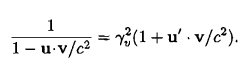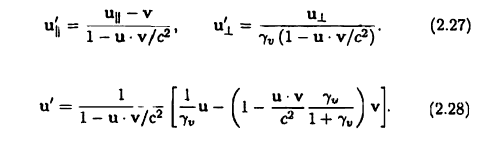# Special Relativity Derivation

Taken from Steane's "Relativity made relatively easy" equation 4.8I have been trying to show (4.8) using these relations earlier on in the book:Tried most means (rearranging, taking dot products) but can't seem to make it work. Is there an easy method I'm missing out?

Last edited:

Dale
Mentor
2021 Award
Try expanding it out in the basis where x is the "u parallel" direction.

Try expanding it out in the basis where x is the "u parallel" direction.
Sorry, I don't understand what you mean by "it". Which term should I expand?

Dale
Mentor
2021 Award
Expand both sides of 4.8.

Expand both sides of 4.8.
I'm trying to derive eq 4.8 starting with the ones below, 2.27 and 2.28.

Dale
Mentor
2021 Award
Then expand all 3. The point is to get them all into the same form.

Then expand all 3. The point is to get them all into the same form.
I wouldn't know what RHS of eq 4.8 would look like initially. I am trying to derive its form starting from 2.27 and 2.28.

jtbell
Mentor
Often, when you want to find a derivation from A to B, it helps to start by working from both A and B to find some equation in the "middle", so to speak. Then you can reverse the steps that took you from B to the "middle." Or, the working might give you enough insight that you can come up with a better way to write the complete derivation.

•Frimus
Often, when you want to find a derivation from A to B, it helps to start by working from both A and B to find some equation in the "middle", so to speak. Then you can reverse the steps that took you from B to the "middle." Or, the working might give you enough insight that you can come up with a better way to write the complete derivation.

Point is, I wouldn't even know what RHS of 4.8 would look like.

OK here is the challenge, rephrased:

Without manipulating RHS of 4.8, use eq 2.27 and 2.28 to show that form.

Dale
Mentor
2021 Award
Without manipulating RHS of 4.8,
Why would you add that restriction?

Just expand it. I don't know why you keep on saying that you don't know what the RHS of 4.8 is. u' and v are vectors, just write them as components and expand in the basis where x is the parallel direction.

I believe unscientific doesn't want to manipulate the RHS of 4.8 because he considers that he isn't supposed to know what it is before deriving it from 2.27 and 2.28 in the first place.

@unscientific: even though you want to derive 4.8 directly from the other two equations, expanding 4.8 will help you understand how you can do that, because some steps may not be obvious. Then you can do the whole derivation starting from 2.27 and 2.28

Ibix
It may help to note that the parallellity (is that a word?) and perpendicularity are with reference to the relative velocity of the moving frame. In that case, u.v=u||×v + u×0.

ChrisVer
Gold Member
Start from:

$\frac{1}{1 - (u \cdot v)/c^2 } = ...$
and you will reach the result. It's straightforward.

Last edited:
Thanks, got it!